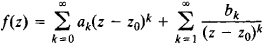# Essential Singularity

Also found in: Wikipedia.

## essential singularity

[i′sen·chəl siŋ·gyə′lar·əd·ē]
(mathematics)
An isolated singularity of a complex function which is neither removable nor a pole.
McGraw-Hill Dictionary of Scientific & Technical Terms, 6E, Copyright © 2003 by The McGraw-Hill Companies, Inc.
The following article is from The Great Soviet Encyclopedia (1979). It might be outdated or ideologically biased.

## Essential Singularity

If a function is single-valued and analytic in some neighborhood of the point z0 in the complex plane and there exists neither a finite nor an infinite limit for the function as zz0, then z0 is said to be an essential singularity of the function (seeANALYTIC FUNCTIONS). For example, the point z = 0 is an essential singularity of such function as e1/z, z sin (1/z), and cos (1/z) + 1n (z + 1).

In a neighborhood of an essential singularity z0, the function f(z) can be expanded in a Laurent series:Here, infinitely many of the numbers b1, b2, ... are nonzero. This property is often used to identify essential singularities.

The behavior of a function in the neighborhood of an essential singularity can be dealt with on the basis of the Casorati-Weier-strass theorem. A generalization of this theorem is provided by Picard’s big, or second, theorem: in every neighborhood of an essential singularity of an analytic function the function takes on every value, with at most one exception. Picard’s theorem has a number of extensions and refinements.

In some branches of the theory of analytic functions, the term “essential singularity” is also applied to singularities of a more complex nature.

### REFERENCES

Markushevich, A. I. Teoriia analiticheskikh funktsii, 2nd ed., vols. 1–2. Moscow, 1967–68.
Nevanlinna, R. Odnoznachnye analiticheskie funktsii. Moscow-Leningrad, 1941. (Translated from German.)